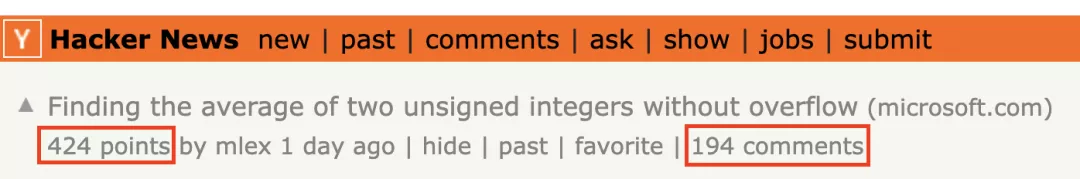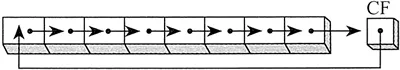There’s a lot to be said for averaging unsigned integers by rounding?

A recent article by Raymond Chen, a Microsoft engineer, has been a direct hit on the technology platform, sparking numerous discussions.Countless people clicked in with unbridled confidence: isn’t it just a simple elementary school programming problem of adding and dividing by two?

 ``````1 2 3 4 `````` ``````unsigned average(unsigned a, unsigned b) { return (a + b) / 2; } ``````

But following deeper, but gradually surprised ……

## Not so simple to find the average

Starting with the method mentioned at the beginning, which any elementary school student would know, this simple method has a fatal flaw.

If the unsigned integers are 32 bits long, then a memory overflow will occur if the two summed values are both half of the maximum length, just in the first step of the summation.

That is, average(0x80000000U, 0x80000000U) = 0.

But there are quite a few solutions, and the first one most experienced developers can think of is to pre-limit the length of the summed numbers to avoid overflow.

There are two specific methods.

1. When the larger of the two unsigned integers is known, subtract the smaller value and divide by two to advance reduce the length :)

 ``````1 2 3 4 `````` ``````unsigned average(unsigned low, unsigned high) { return low + (high - low) / 2; } ``````
2. Pre-divide two unsigned integers while correcting the lower digit by & to ensure that the result is still correct when both integers are odd.

(Incidentally, this is a method that was patented and expired in 2016)

 ``````1 2 3 4 `````` ``````unsigned average(unsigned a, unsigned b) { return (a / 2) + (b / 2) + (a & b & 1); } ``````

Both of these are more common ideas, and many users also said that the fastest they could think of was 2016 patent method .

The same method that can be quickly thought of by the majority of users is also SWAR (SIMD within a register).

 ``````1 2 3 4 `````` ``````unsigned average(unsigned a, unsigned b) { return (a & b) + (a ^ b) / 2;// 变体 (a ^ b) + (a & b) * 2 } ``````

and the `std: : midpoint` function in C++ version 20.

Next, the authors propose a second idea.

If the unsigned integer is 32 bits and the native register size is 64 bits, or if the compiler supports multi-word operations, the summed value can be forced into long integer data.

 ``````1 2 3 4 5 6 `````` ``````unsigned average(unsigned a, unsigned b) { // Suppose "unsigned" is a 32-bit type and // "unsigned long long" is a 64-bit type. return ((unsigned long long)a + b) / 2; } ``````

However, there is one particular point to note here.

You must ensure that the first 32 bits of the 64-bit register are all zeros in order not to affect the remaining 32-bit values.

Architectures such as x86-64 and aarch64 automatically extend 32-bit values zero to 64-bit values.

 `````` 1 2 3 4 5 6 7 8 9 10 11 12 13 14 15 `````` ``````// x86-64: Assume ecx = a, edx = b, upper 32 bits unknown mov eax, ecx ; rax = ecx zero-extended to 64-bit value mov edx, edx ; rdx = edx zero-extended to 64-bit value add rax, rdx ; 64-bit addition: rax = rax + rdx shr rax, 1 ; 64-bit shift: rax = rax >> 1 ; result is zero-extended ; Answer in eax // AArch64 (ARM 64-bit): Assume w0 = a, w1 = b, upper 32 bits unknown uxtw x0, w0 ; x0 = w0 zero-extended to 64-bit value uxtw x1, w1 ; x1 = w1 zero-extended to 64-bit value add x0, x1 ; 64-bit addition: x0 = x0 + x1 ubfx x0, x0, 1, 32 ; Extract bits 1 through 32 from result ; (shift + zero-extend in one instruction) ; Answer in x0 ``````

In contrast, architectures such as Alpha AXP and mips64 extend 32-bit values symbols to 64-bit values.

At such times, it is necessary to add additional instructions to return to zero, for example, through the delete instruction rldicl that rounds two words to the left.

 `````` 1 2 3 4 5 6 7 8 9 10 11 12 13 14 15 16 17 18 19 20 21 `````` ``````// Alpha AXP: Assume a0 = a, a1 = b, both in canonical form insll a0, #0, a0 ; a0 = a0 zero-extended to 64-bit value insll a1, #0, a1 ; a1 = a1 zero-extended to 64-bit value addq a0, a1, v0 ; 64-bit addition: v0 = a0 + a1 srl v0, #1, v0 ; 64-bit shift: v0 = v0 >> 1 addl zero, v0, v0 ; Force canonical form ; Answer in v0 // MIPS64: Assume a0 = a, a1 = b, sign-extended dext a0, a0, 0, 32 ; Zero-extend a0 to 64-bit value dext a1, a1, 0, 32 ; Zero-extend a1 to 64-bit value daddu v0, a0, a1 ; 64-bit addition: v0 = a0 + a1 dsrl v0, v0, #1 ; 64-bit shift: v0 = v0 >> 1 sll v0, #0, v0 ; Sign-extend result ; Answer in v0 // Power64: Assume r3 = a, r4 = b, zero-extended add r3, r3, r4 ; 64-bit addition: r3 = r3 + r4 rldicl r3, r3, 63, 32 ; Extract bits 63 through 32 from result ; (shift + zero-extend in one instruction) ; result in r3 ``````

Or directly access SIMD registers that are larger than the native registers. Of course, crossing from general-purpose registers to SIMD registers will certainly increase memory consumption as well.

If the computer’s processor supports rounding addition, then a third idea can also be used.

In this case, if the register size is n bits, then the sum of two n-bit unsigned integers can be interpreted as n+1 bits, and by using the RCR (cyclic right shift with rounding) instruction, the correct average value can be obtained without losing the overflowing bits.`````` 1 2 3 4 5 6 7 8 9 10 11 12 13 14 15 16 17 18 19 20 `````` ``````// x86-32 mov eax, a add eax, b ; Add, overflow goes into carry bit rcr eax, 1 ; Rotate right one place through carry // x86-64 mov rax, a add rax, b ; Add, overflow goes into carry bit rcr rax, 1 ; Rotate right one place through carry // 32-bit ARM (A32) mov r0, a adds r0, b ; Add, overflow goes into carry bit rrx r0 ; Rotate right one place through carry // SH-3 clrt ; Clear T flag mov a, r0 addc b, r0 ; r0 = r0 + b + T, overflow goes into T bit rotcr r0 ; Rotate right one place through carry ``````

What if the processor does not support right-shift operations with a round-robin?

You can also use the inner loop (rotation intrinsic).

 `````` 1 2 3 4 5 6 7 8 9 10 11 12 13 14 15 16 `````` ``````unsigned average(unsigned a, unsigned b) { #if defined(_MSC_VER) unsigned sum; auto carry = _addcarry_u32(0, a, b, &sum); sum = (sum & ~1) | carry; return _rotr(sum, 1); #elif defined(__clang__) unsigned carry; sum = (sum & ~1) | carry; auto sum = __builtin_addc(a, b, 0, &carry); return __builtin_rotateright32(sum, 1); #else #error Unsupported compiler. #endif } ``````

The result is that code generation for the x86 architecture has not changed much, code generation for the MSCver architecture has gotten worse, and code generation for the arm-thumb2 clang has gotten better.

 `````` 1 2 3 4 5 6 7 8 9 10 11 12 13 14 15 16 17 18 19 20 21 22 23 `````` ``````// _MSC_VER mov ecx, a add ecx, b ; Add, overflow goes into carry bit setc al ; al = 1 if carry set and ecx, -2 ; Clear bottom bit movzx ecx, al ; Zero-extend byte to 32-bit value or eax, ecx ; Combine ror ear, 1 ; Rotate right one position ; Result in eax // __clang__ mov ecx, a add ecx, b ; Add, overflow goes into carry bit setc al ; al = 1 if carry set shld eax, ecx, 31 ; Shift left 64-bit value // __clang__ with ARM-Thumb2 movs r2, #0 ; Prepare to receive carry adds r0, r0, r1 ; Calculate sum with flags adcs r2, r2 ; r2 holds carry lsrs r0, r0, #1 ; Shift sum right one position lsls r1, r2, #31 ; Move carry to bit 31 adds r0, r1, r0 ; Combine ``````

## Reflections of a Microsoft Engineer

Raymond Chen joined Microsoft in 1992 and has served for 25 years so far, doing UEX-Shell and also participating in Windows development, and he did much of the initial UI architecture for Windows.His blog The Old New Thing on MSDN is also very well known in the industry as a purely technical output site.

The comments section of this blog is also infested with Microsoft experts from all walks of life, and continues to delve deeper.

A new approach has been proposed, with a total of 36 loops in the MIPS ASM.

 `````` 1 2 3 4 5 6 7 8 9 10 11 12 13 `````` ``````unsigned avg(unsigned a, unsigned b) { return (a & b) + (a ^ b) / 2; } // lw \$3,8(\$fp) # 5 // lw \$2,12(\$fp) # 5 // and \$3,\$3,\$2 # 4 // lw \$4,8(\$fp) # 5 // lw \$2,12(\$fp) # 5 // xor \$2,\$4,\$2 # 4 // srl \$2,\$2,1 # 4 // addu \$2,\$3,\$2 # 4 ``````

In response to the 2016 patent law, someone said that instead of using (a / 2) + (b / 2) + (a & b & 1), why not just put (a & 1) & ( b & 1 ) ) into the adder as an integer?

Someone in the comments section also recommended the TopSpeed compiler, which can define an inline function by specifying the appropriate code bytes and calling conventions to solve the “multiply and divide result is 16 bits, but the middle calculated value is not” scenario.

Let’s just say that there is no end to learning.